# VBA Sleep FunctionArticle byExcelMojo Team## What is Excel VBA Sleep Function?

VBA Sleep function is a windows function in VBA that pauses certain macro actions with specified time intervals. After the specified time, we can resume the macro and complete the remaining portion of the code.

Sometimes we may have to pause the running macro to complete specific tasks and resume it. In such cases, the VBA Sleep function helps us pause the macro and allows us to perform additional tasks in between.

The above code will start the macro function, wait for 10 seconds, and then resumes it. When we initiate the macro, the time is as follows.

Once we click on OK, it will go to sleep for 10 seconds and the end time will be as follows.

The difference between the start and end times is precisely 10 seconds, and here we can perform any other supporting task.

Code:

Sub Sleep_Intro()
Dim ST As String
Dim ET As String
ST = Time
MsgBox ST
Sleep (10000)
ET = Time
MsgBox ET
End Sub

###### Key Takeaways
• VBA Sleep Function is used to pause the macro for a specified time period.
• We need to call the API code at the top of the module to access the VBA Sleep function.
• Sleep function accepts the time in milliseconds, i.e., 1000 milliseconds is equal to 1 second; similarly, 10000 milliseconds equals 10 seconds.
• We can identify the time a macro has paused using the TIMER function by capturing the start time at the top and end time at the end of the macro.

### What Does Sleep Function Do?

As the name suggests, VBA Sleep makes the macro ‘sleep’ for a specified period. Sleep is not a built-in function in VBA because it is a Windows function.

We must enter the specified set of codes to call this function in VBA. The VBA Sleep function is available inside the Windows DLL files; hence, the API nomenclature must be declared before we start the macro subroutine.

The following code should be entered at the top of the module to call the VBA Sleep function.

#If VBA7 Then
Public Declare PtrSafe Sub Sleep Lib “kernel32” (ByVal dwMilliseconds As LongPtr)
‘For sleep function 64 bit versions of Excel
#Else
Public Declare Sub Sleep Lib “kernel32” (ByVal dwMilliseconds As Long)
‘For sleep function 32 bit versions of Excel
#End If

Copy the above API calling code and paste it at the top of the visual basic editor module. The following image is a reference for you.

Once we enter the above code, we can call the VBA Sleep function.

Before we go into the example of using the VBA Sleep function, let us give you a little background on the Sleep function terminologies.

Using the VBA Sleep function, we can delay the macro for milliseconds. For example, 1000 milliseconds equals 1 second; on similar lines, 10000 milliseconds equals 10 seconds.

Follow the steps below to write the VBA Sleep function code from scratch.

1. After you copy and paste the API code at the top of the module, create a sub-routine procedure by naming the macro.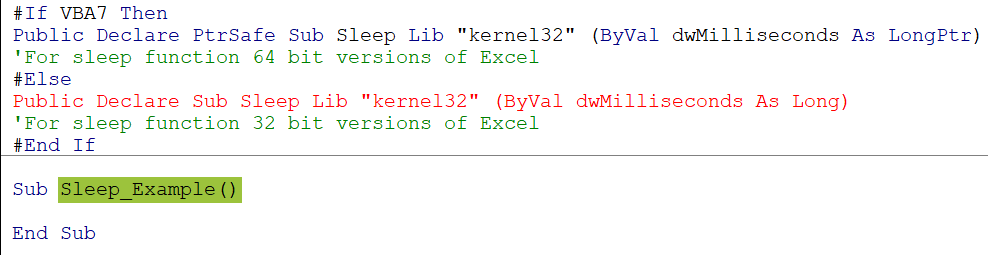2. Define a variable with string data type to get the start time of the VBA sleep function.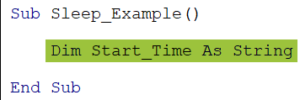3. Define another variable to get the end time with the string data type.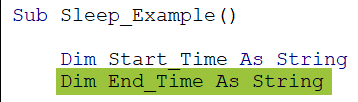4. Now, for the Start_Time variable assign the starting time by using the TIME property.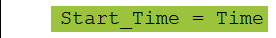Note: TIME is a property available in VBA to capture the current time per the system. It is like the NOW function, which gives us the current date and time based on our system.

5. Now let’s show the starting time in a message box.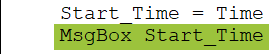6. Now enter the SLEEP function.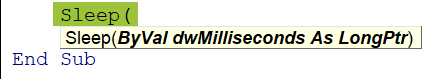7. Assume we need to pause the macro for 5 seconds, then enter the required number of seconds in milliseconds i.e., as mentioned, we told earlier 5000 milliseconds is equal to 5 seconds hence enter 5000 inside the Sleep function.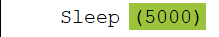8. Now for the End_Time variable again assign the TIME property to get the current time after the sleep function paused the macro for 5 seconds.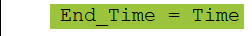9. Now show the end timing in a message box.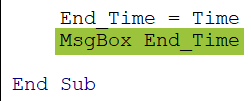To understand the VBA sleep function macro code, we need to execute the code line by line by pressing the F8 key. F8 key will execute the 1 line at a time, press F8 key and it will start the macro.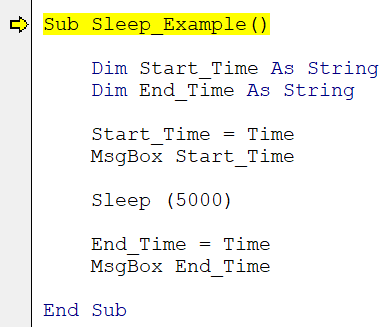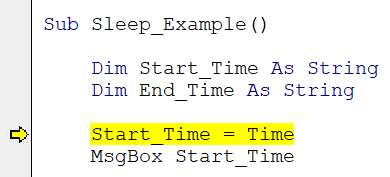The yellow highlighted line is not yet executed; upon pressing the F8 key again, it will execute that line and capture the start time in the message box.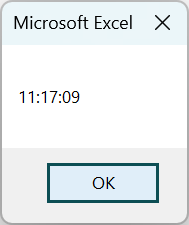The start time is 11:17:09 and then VBA sleep function will pause the macro for 5 second, and we will see the end time as below.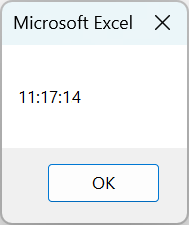The macro started at the 09th second, and then the VBA Sleep function paused the macro for 5 seconds; hence the end time shows as 14, i.e., 5 second gap between the start and end time.

Code:

Sub Sleep_Example()
Dim Start_Time As String
Dim End_Time As String
Start_Time = Time
MsgBox Start_Time
Sleep (5000)
End_Time = Time
MsgBox End_Time
End Sub

### Examples

Let’s look at some of the VBA Sleep function examples.

#### Example #1 – Rename Sheet and Pause Macro Between Sheets

For example, look at the following Excel workbook containing two sheets.

We have two worksheets named Intro and Basic, respectively. Assume we must rename the “Intro” sheet to Introduction and the “Basic” sheet to Basics.

While we rename the sheet, we will apply the Sleep function between the two sheets’ activity and pause the macro for 10 seconds.

The following code is for your reference.

Code Explanation:

• Part #1: Here, we have defined two variables with a worksheet object reference. For these two variables we will set the two worksheet references.
• Part #2: We are setting the required worksheet’s reference for the defined variables. Ws1 will have the “Intro” worksheet reference, while Ws2 will have the “Basic” worksheet reference.
• Part #3: We are renaming the worksheet “Intro” to “Introduction”.
• Part #4: We have applied the Sleep function with a 10-second interval time. Before we use the Sleep function, a message box will be displayed saying, “Now macro will be paused for 10 seconds.”.
• Part #5: After the 10-second break, we rename the worksheet “Basic” to “Basics.” By pressing the F5 function key, let’s execute the code.

Upon pressing the F5 key “Intro” sheet renamed to “Introduction,” and we will see a message box alerting us about the coming sleep function.

Click on the “Ok” button of the message box, and the Sleep function will pause the macro for 10 seconds. Then, we will see the “Basic” worksheet renamed “Basics.”

After the 10 second pause macro resumed and renamed the Basic sheet to Basics.

Code:

Sub Sleep_Rename()
Dim Ws1 As Worksheet
Dim Ws2 As Worksheet
Set Ws1 = Worksheets(“Intro”)
Set Ws2 = Worksheets(“Basic”)
Ws1.Name = “Introduction”
MsgBox “Now macro will be paused for 10 seconds”
Sleep (10000)
Ws2.Name = “Basics”
End Sub

#### Example #2 – Sleep Function in Loops

VBA Sleep function can be used with VBA loops where we can pause the macro within the loop for a specified amount of time. For example, assume we must insert serial numbers from 1 to 10. Without the Sleep function, we can achieve this quickly.

The following code will insert serial numbers from 1 to 10 without the Sleep function, and we can see the total time taken to run the macro.

When we run this macro, it will insert the serial numbers from 1 to 10 and show the total time taken to complete the macro at the end. Here, it is 3.01 seconds.

It has inserted serial numbers from 1 to 10 and it shows the total time taken to complete the macro as 3.01 seconds.

Now, let’s add the Sleep function inside the loop.

We have applied the Sleep function inside, and the loop waits for 3 seconds every time the code runs inside the loop. Now, let’s run the code and see what happens.

Loop ran ten times (1 to 10), and the macro paused for 3 seconds each time. Hence, the total time taken to complete the macro is 30.11 seconds.

Code:

Sub Sleep_Loops()
Dim Start_Time As Double
Dim End_Time As Double
Start_Time = Timer
Dim k As Long
For k = 1 To 10
Cells(k, 1).Value = k
Sleep (3000)
Next k
End_Time = Timer
Dim Total_Time As Variant
Total_Time = Round(End_Time – Start_Time, 2)
MsgBox “Total time taken is ” & Total_Time & ” Seconds”
End Sub

### Important Things to Note

• The VBA Sleep Function is not a built-in function. Hence, we must wait to access this. Instead, we must use the API code (given in this article) at the top of the module.
• If the VBA Sleep Function is not defined, we cannot access the Sleep function.
• VBA Sleep Function 64 bit has different API code and 32-bit has different API code.
• VBA Sleep Function accepts only numerical values.

1. What is the difference between Wait and Sleep in Excel VBA?

Both Wait and Sleep functions in Excel VBA allows us to pause the macro execution for specified time to carry out other events or tasks. However, there are some differences between these two, and those are;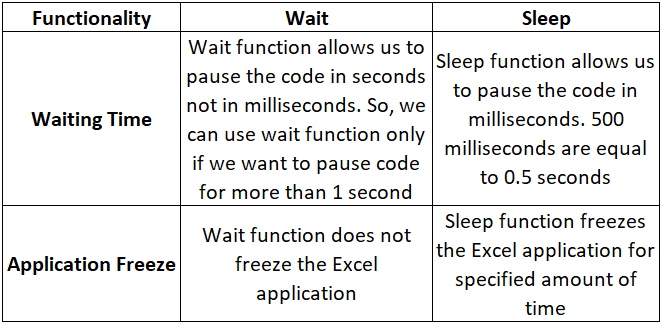2. Why is Sleep function in Excel VBA not working?

Sleep function in VBA will not work for two reasons and those are as below.
We need to first define the API code at the top of the module; otherwise, we cannot access the VBA Sleep function. If the vba sleep function not defined, then we will end up getting the error.
We must give the pause time in milliseconds. Hence, the Sleep function input must be a numerical value. Anything other than numerical value will throw an error.

3. What is the alternative to Sleep in VBA?

An alternative to sleep function is “Do Events” and Wait method in VBA.
• Wait: This allows us to pause the code execution for specific amounts of time.
• Do Events: This will help us to do certain things inside the loop.